Evaluating Functions

Functions can be evaluated on the graphing calculator by
the use of functional notation or by graphing.

1.
 Evaluating functions using functional notation.

If f (x) = 3x3 + 2x - 5,  find  f (23.6).  Round to 3 decimal places, if needed.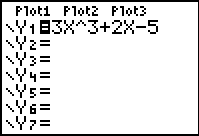Enter the function in Y=.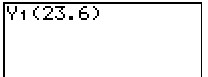Go to the home screen.  Using a functional notation format, enter Y1(23.6).  [To get Y1, go to VARS, arrow right to Y-VARS, #1Function, #1Y1.]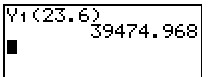Hit ENTER. Answer:  39,474.968

Examples:

 Function: Evaluate: Answer (round to 3 decimal places if needed) 1.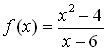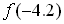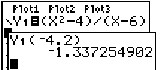Answer:  -1.337 2.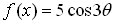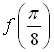Set MODE to Radian.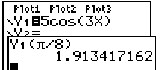Answer:  1.913 3.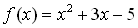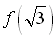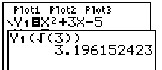Answer:  3.196

2.
 Evaluating functions by graphing.

If f (x) = 3x3 + 2x - 5,  find  f (23.6).  Round to 3 decimal places, if needed.
 Step 1: Step 2: Step 3:Enter the function in Y=.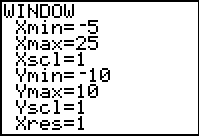Adjust the WINDOW so that the point 23.6 will be shown on the x-axis.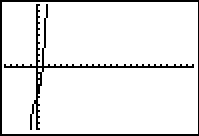Graph Step 4: Step 5: Step 6: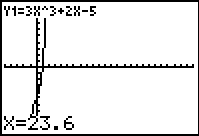Hit TRACE.  Type 23.6 right on the screen.  It will automatically appear at the bottom of the graph.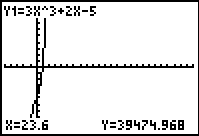Hit ENTER. The answer (the y-value) will appear. Answer:  39,474.068